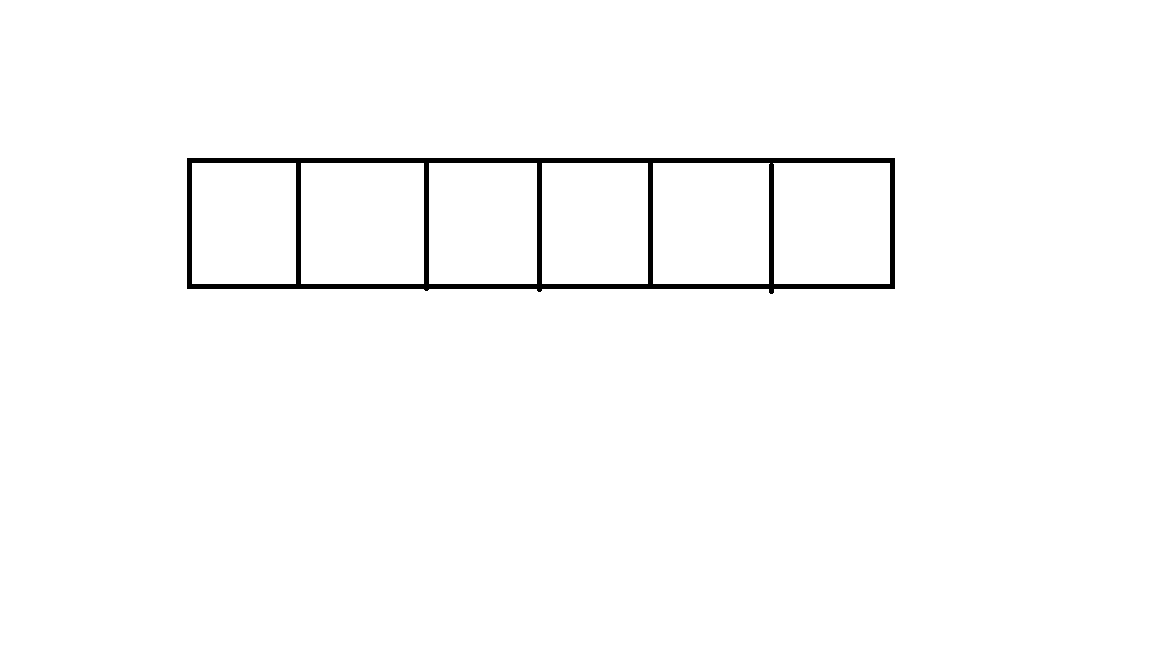# 欢迎来到Nepire的校OJ入门题解——————蓝桥选拔篇（六）

Description

Input

Output

Sample Input

2
2 3
4 6
3 4
1 2 1

Sample Output

-1
1

Source

### 解题思路### 参考代码

#include <ctype.h>
#include <errno.h>
#include <float.h>
#include <stdio.h>
#include <stdlib.h>
#include <string.h>
#include <time.h>
#include <wchar.h>
#include <wctype.h>
#include <assert.h>
#include <limits.h>
#include <locale.h>
#include <math.h>
#include <stdio.h>
#define m 1001
int i,t,n,k,a[m],sum=0,ave=0,ans,yy,j,suma;
int main()
{
scanf("%d",&t);
while(t--)
{
ans=0;
sum=0;
yy=0;
scanf("%d%d",&n,&k);
for(i=0;i<n;i++)
{
scanf("%d",&a[i]);
sum += a[i];
}

if(sum%k != 0)
{
printf("-1\n");
}
else
{
ave=sum/k;
ans=n+k-2;
for(i=0;i<k-1;i++)
{
yy += ave;

for(j=0;j<n-1;j++)
{
suma += a[j];
if(suma==yy)
{
ans -= 2;
}

}
suma=0;//suma??0

}
printf("%d\n",ans);
}

}
return 0;
}

### ——Nepire

12-17302
12-1738712-15281
12-06262
12-07320
12-15287
12-17586
12-01509
12-18648
12-21105